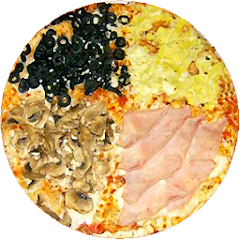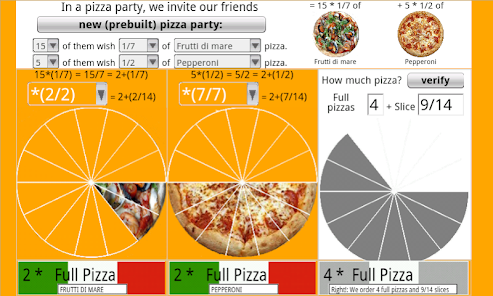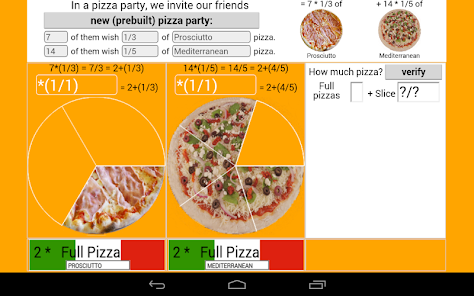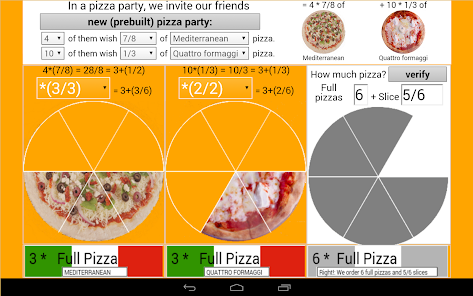# How much pizza to order?

1K+EveryoneAndroid version of the "How Much Pizza to order?
http://www.nummolt.com/obbl/howmuchpizza/index.html

"How much pizza...?" About adding fractions: Working with Improper fractions and Mixed fractions. The program build the problem, and you can solve filling the textboxes containing question marks. There's a tool that helps you find the common denominator.

Nummolt - OBBL Math Toys
http://www.nummolt.com/obbl/index.html

MathTools reference:
http://mathforum.org/mathtools/tool/186520/

Math 3 Equivalent fractions
Math 4 Equivalent fractions, Simplifying fractions, Adding fractions, Fractions <--> decimals
Math 5, 6, 7 Equivalent fractions, Simplifying fractions, Adding fractions, Multiplying fractions, Writing decimals, Fractions <--> decimals

For more difficult exercises on fractions, we recommend:
Mathcats: "Old Egyptian Fractions":
Updated on
Oct 5, 2020

## Data safety

Safety starts with understanding how developers collect and share your data. Data privacy and security practices may vary based on your use, region, and age. The developer provided this information and may update it over time.No data shared with third parties